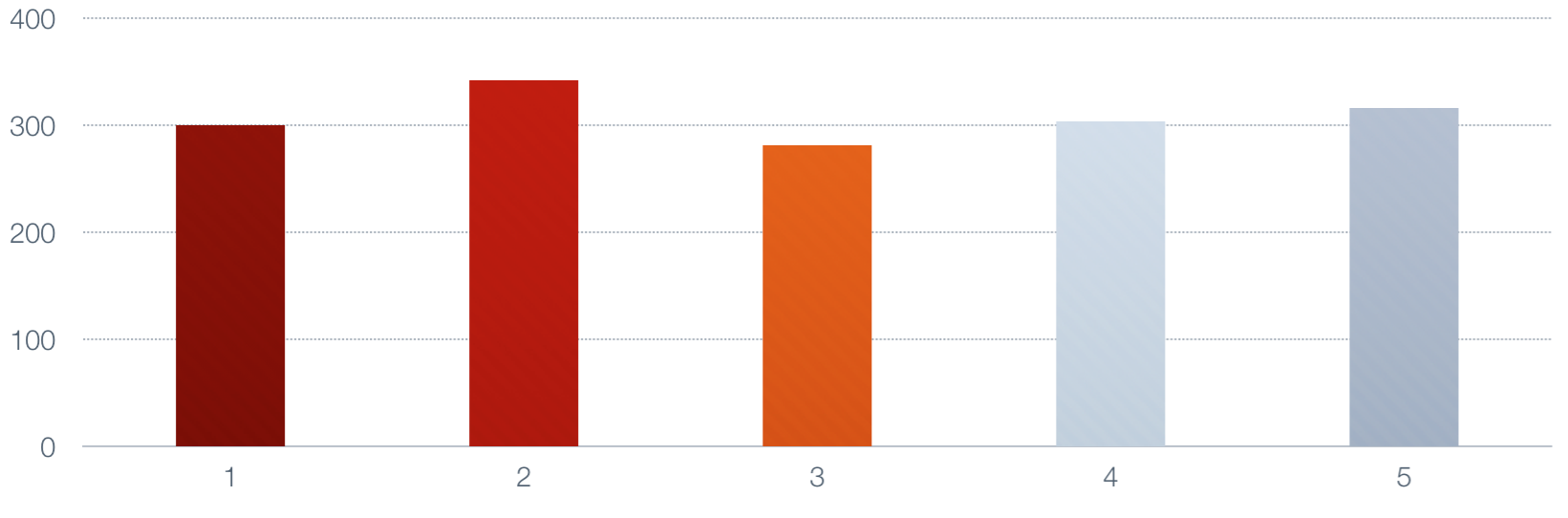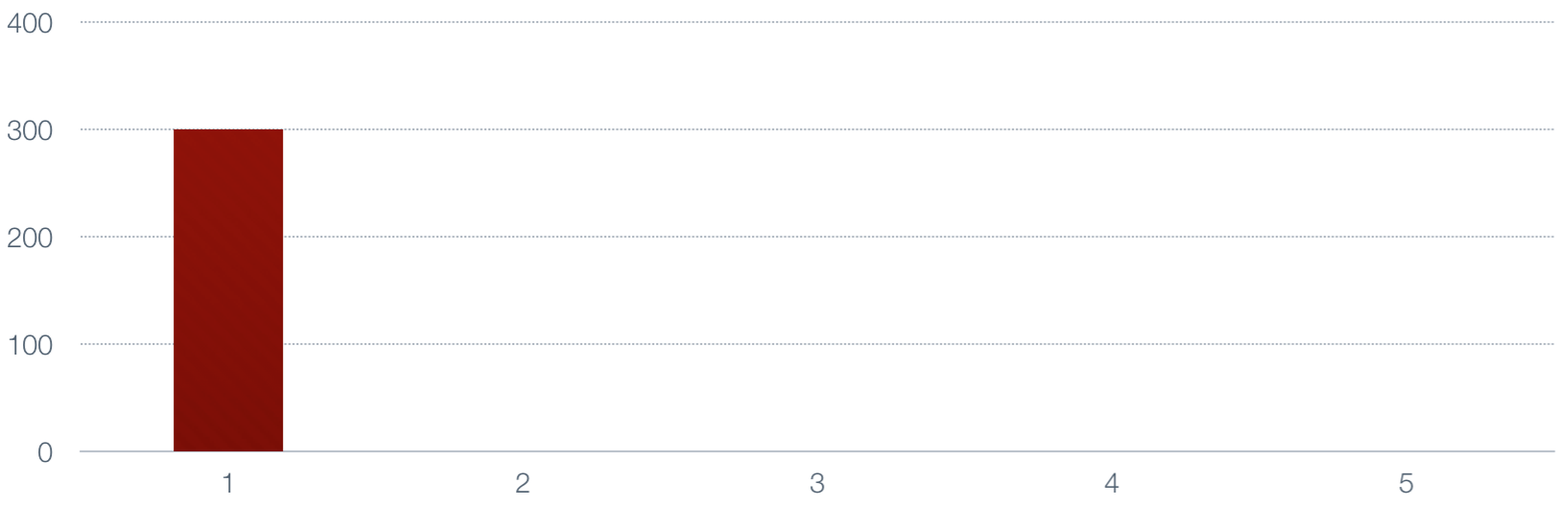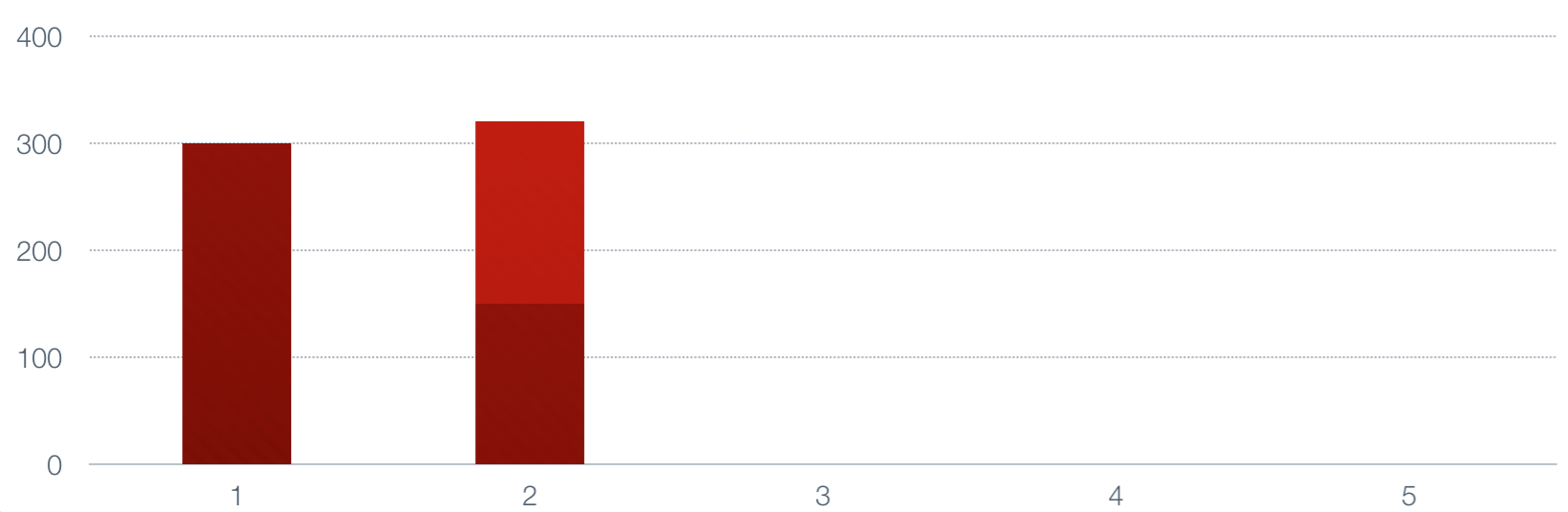# VividCortex/ewma

Exponentially Weighted Moving Average algorithms for Go.
Latest commit 43880d2 Aug 4, 2017
Type Name Latest commit message Commit time
Failed to load latest commit information..github.gitignore Jul 8, 2013LICENSEREADME.mdewma.go Jun 29, 2016ewma_test.go Jul 27, 2017

# EWMAThis repo provides Exponentially Weighted Moving Average algorithms, or EWMAs for short, based on our Quantifying Abnormal Behavior talk.

### Exponentially Weighted Moving Average

An exponentially weighted moving average is a way to continuously compute a type of average for a series of numbers, as the numbers arrive. After a value in the series is added to the average, its weight in the average decreases exponentially over time. This biases the average towards more recent data. EWMAs are useful for several reasons, chiefly their inexpensive computational and memory cost, as well as the fact that they represent the recent central tendency of the series of values.

The EWMA algorithm requires a decay factor, alpha. The larger the alpha, the more the average is biased towards recent history. The alpha must be between 0 and 1, and is typically a fairly small number, such as 0.04. We will discuss the choice of alpha later.

The algorithm works thus, in pseudocode:

1. Multiply the next number in the series by alpha.
2. Multiply the current value of the average by 1 minus alpha.
3. Add the result of steps 1 and 2, and store it as the new current value of the average.
4. Repeat for each number in the series.

There are special-case behaviors for how to initialize the current value, and these vary between implementations. One approach is to start with the first value in the series; another is to average the first 10 or so values in the series using an arithmetic average, and then begin the incremental updating of the average. Each method has pros and cons.

It may help to look at it pictorially. Suppose the series has five numbers, and we choose alpha to be 0.50 for simplicity. Here's the series, with numbers in the neighborhood of 300.Now let's take the moving average of those numbers. First we set the average to the value of the first number.Next we multiply the next number by alpha, multiply the current value by 1-alpha, and add them to generate a new value.This continues until we are done.Notice how each of the values in the series decays by half each time a new value is added, and the top of the bars in the lower portion of the image represents the size of the moving average. It is a smoothed, or low-pass, average of the original series.

For further reading, see Exponentially weighted moving average on wikipedia.

### Choosing Alpha

Consider a fixed-size sliding-window moving average (not an exponentially weighted moving average) that averages over the previous N samples. What is the average age of each sample? It is N/2.

Now suppose that you wish to construct a EWMA whose samples have the same average age. The formula to compute the alpha required for this is: alpha = 2/(N+1). Proof is in the book "Production and Operations Analysis" by Steven Nahmias.

So, for example, if you have a time-series with samples once per second, and you want to get the moving average over the previous minute, you should use an alpha of .032786885. This, by the way, is the constant alpha used for this repository's SimpleEWMA.

### Implementations

This repository contains two implementations of the EWMA algorithm, with different properties.

The implementations all conform to the MovingAverage interface, and the constructor returns that type.

Current implementations assume an implicit time interval of 1.0 between every sample added. That is, the passage of time is treated as though it's the same as the arrival of samples. If you need time-based decay when samples are not arriving precisely at set intervals, then this package will not support your needs at present.

#### SimpleEWMA

A SimpleEWMA is designed for low CPU and memory consumption. It will have different behavior than the VariableEWMA for multiple reasons. It has no warm-up period and it uses a constant decay. These properties let it use less memory. It will also behave differently when it's equal to zero, which is assumed to mean uninitialized, so if a value is likely to actually become zero over time, then any non-zero value will cause a sharp jump instead of a small change.

#### VariableEWMA

Unlike SimpleEWMA, this supports a custom age which must be stored, and thus uses more memory. It also has a "warmup" time when you start adding values to it. It will report a value of 0.0 until you have added the required number of samples to it. It uses some memory to store the number of samples added to it. As a result it uses a little over twice the memory of SimpleEWMA.

## Usage

### API Documentation

View the GoDoc generated documentation here.

```package main

import "github.com/VividCortex/ewma"

func main() {
samples := float64{
4599, 5711, 4746, 4621, 5037, 4218, 4925, 4281, 5207, 5203, 5594, 5149,
}

e := ewma.NewMovingAverage()  //=> Returns a SimpleEWMA if called without params
a := ewma.NewMovingAverage(5) //=> returns a VariableEWMA with a decay of 2 / (5 + 1)

for _, f := range samples {
}

e.Value() //=> 13.577404704631077
a.Value() //=> 1.5806140565521463e-12
}```

## Contributing

We only accept pull requests for minor fixes or improvements. This includes:

• Small bug fixes
• Typos
• Documentation or comments

Please open issues to discuss new features. Pull requests for new features will be rejected, so we recommend forking the repository and making changes in your fork for your use case.